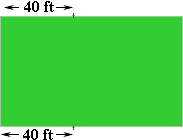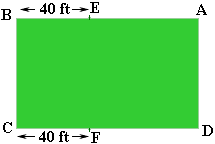Quandaries and Queries Who is asking: Parent Level: All Question: A rectangular corral is forty feet longer than it is wide. If the perimeter is 220 feet, determine the length and width of the corral. Hi, I would draw a diagram. This is the diagram I drew.I need to put some labels on the diagram so that I can tell you how it helped me solve the problem.Since the corral is 40 feet longer than it is wide I put a point E on the side AB so that the length of AE is the same as the length AD. Thus the length of BE is 40 feet. In a similar fashion I put the point F on CD so that the length of CF is 40 feet. Thus the lengths of AE, BC, FD and AD are all the same. If I just knew that length I would know the perimeter. To set up an equation let this unknown length be x feet. Then I know that perimeter = 220 feet = |AE| + 40 + |BC| + 40 + |FD| + |DA| = x + 40 + x + 40 + x + x = 4x + 80 Thus 4x + 80 = 220 Now solve for x, which is the width of the corral. Cheers, Penny Go to Math Central